# Reverse function

The concept of inverse functions: Let the functiontakes every value in a single point in its domain (such a function is called invertible ). Then for each number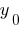( from the set of function values) there is a single value( scope function), such that,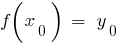. Consider the functionthat to each numberput in the number, that is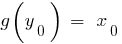. In this case, the functionis called inverse function.

## Properties of inverse functions

1. Scope direct function is the set of values of the reverse, and the set of values of the direct function, the scope back.
2.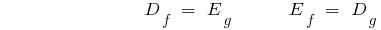3. If the function is increasing (decreasing) on some interval, then it has an inverse function on this interval, which increases if the direct function is increasing and decreasing, if the video feature comes in.
4. Graphs of direct and inverse functions are symmetrical with respect to the line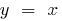(the bisector of the first and third coordinate angles)

## Examples of inverse functions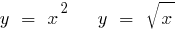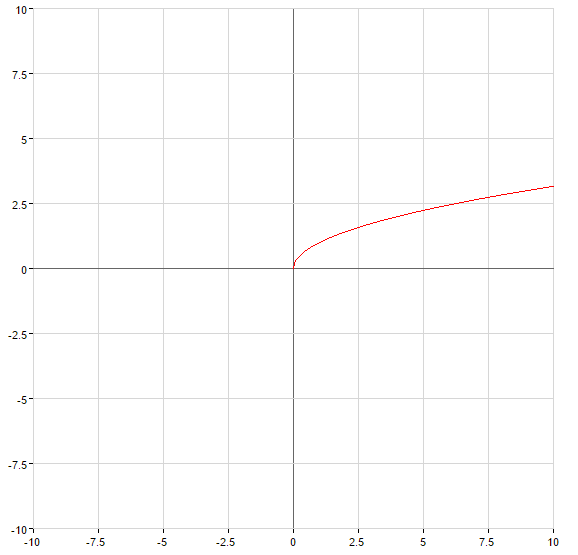## An example of finding inverse functions

Example: to Find the inverse function for the function: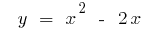Solution: Find where the given function is increasing and decreasing. Then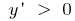when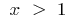a function is increasing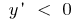whenthe function decreases.

At each of these intervalsand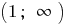write the formula of the inverse function. Since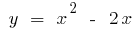then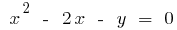.

Hence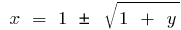, that is when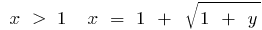, and when. Changing the symbols on the traditional, we get: for a functionwithinverse function is a function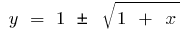, whilethe inverse function is a function.

Tags:
Chapter:
Versions in other languages:
Share with friends: